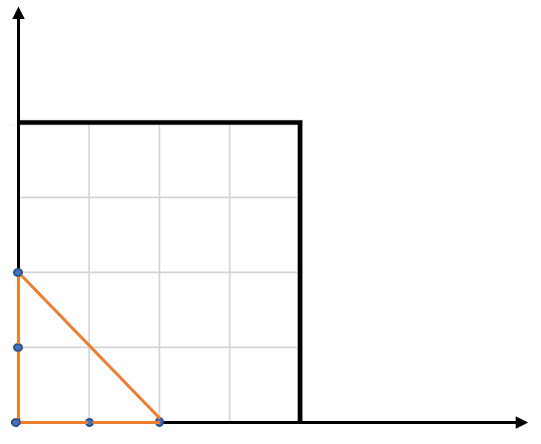# GeetCode Hub

You have a list of points in the plane. Return the area of the largest triangle that can be formed by any 3 of the points.

```Example:
Input: points = [[0,0],[0,1],[1,0],[0,2],[2,0]]
Output: 2
Explanation:
The five points are show in the figure below. The red triangle is the largest.
```Notes:

• `3 <= points.length <= 50`.
• No points will be duplicated.
•  `-50 <= points[i][j] <= 50`.
• Answers within `10^-6` of the true value will be accepted as correct.

public class Solution { public double LargestTriangleArea(int[][] points) { } }